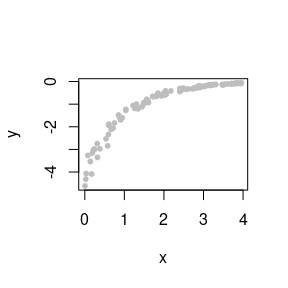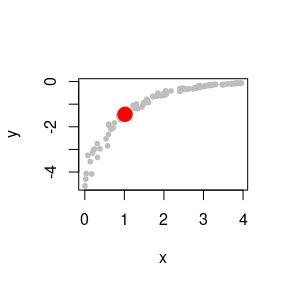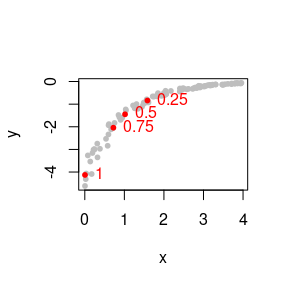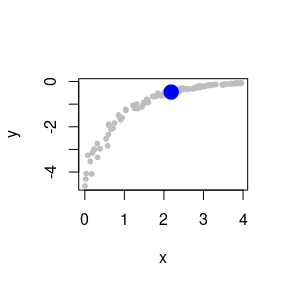# Input data

First we need to load the package and load the coordinates of points that define a knee curve. Here is a randomly-generated example of a knee curve:

library(KneeArrower)

set.seed(12345)
x <- runif(100, min=0, max=4)
y <- -exp(-x) * (1+rnorm(100) * 0.10) * 4
plot(x, y, pch=20, col="gray")Knee curves can be either increasing or decreasing, concave up or concave down.

# Finding cutoff points

Use the findCutoff function to find cutoff points on the curve using the first derivative cutoff method or the maximum curvature method.

Note that cutoff points aren’t exact because the derivatives have to be estimated using curve fitting.

## First derivative cutoff

This method finds the point along the curve where the slope is a given fraction of the maximum. This is the default method.

For example, here is the point at which the slope of tangent line is half of its maximum value.

cutoff.point <- findCutoff(x, y, method="first", 0.5)
cutoff.point
## $x ##  1.017362 ## ##$y
##  -1.448078
plot(x, y, pch=20, col="gray")
points(cutoff.point, col="red", cex=3, pch=20)You can set cutoffs higher or lower on the curve by setting the first derivative cutoff to different values between 0 and 1.

thresholds <- c(0.25, 0.5, 0.75, 1)

# Find cutoff points at each threshold
cutoff.points <- lapply(thresholds, function(i) {
findCutoff(x, y, method="first", i)
})
x.coord <- sapply(cutoff.points, function(p) p$x) y.coord <- sapply(cutoff.points, function(p) p$y)

# Plot the cutoff points on the scatterplot
plot(x, y, pch=20, col="gray")
points(x.coord, y.coord, col="red", pch=20)
text(x.coord, y.coord, labels=thresholds, pos=4, col="red")## Maximum curvature cutoff

This method finds the point at which the circle tangent to the curve has the smallest radius.

cutoff.point <- findCutoff(x, y, method="curvature")
cutoff.point
## $x ##  2.183521 ## ##$y
##  -0.4642701
plot(x, y, pch=20, col="gray")
points(cutoff.point, col="blue", cex=3, pch=20)